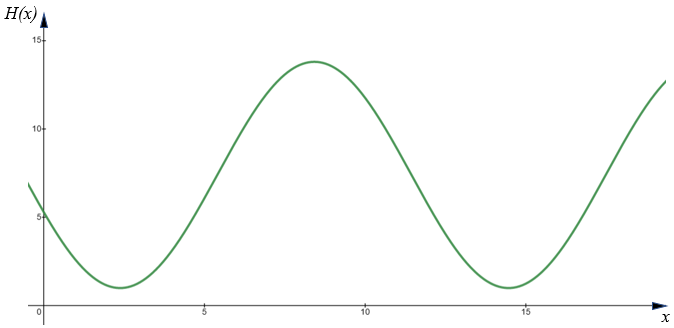# Exam-Style Question on Circular functions

## A mathematics exam-style question with a worked solution that can be revealed gradually

##### List Of QuestionsExam-Style QuestionMore Circular QuestionsMore on this Topic

Question id: 618. This question is similar to one that appeared on an IB AA Standard paper in 2021. The use of a calculator is allowed.

The height of water in metres above Chart Datum* in the Port of Bristol on a day in January is modelled by the function:

$$H(x) = a \sin(b(x-c)) +d$$

where $$x$$ is the number of hours after midnight GMT, and $$a,b,c, \text{ and } d$$ are constants greater than zero.

The following graph shows the height of the water for 19 hours starting at midnight.The first low tide occurs at 02:23 and next low tide occurs at 14:28.

Throughout the day the height of the water fluctuates between 1m and 13.8m.

(a) Show that $$a = 6.4$$.

(b) Find the value of $$b$$ to two decimal places.

(c) Find the value of $$d$$.

(d) Find the smallest possible value of $$c$$ to one decimal place.

(e) Using the values of $$a,b,c, \text{ and } d$$ found above, find the height of the water at 10:00.

(f) Determine the number of hours, during this January day, for which the tide is higher than 10 metres above Chart Datum.

* Chart Datum is a reference datum or level from which heights and depths on a nautical chart are measured. Chart Datum is determined by the lowest astronomical tide, which is the level of the sea at its lowest possible level. It is also the reference level used to measure the depth of water in a harbour or other body of water.

 The worked solutions to these exam-style questions are only available to those who have a Transum Subscription. Subscribers can drag down the panel to reveal the solution line by line. This is a very helpful strategy for the student who does not know how to do the question but given a clue, a peep at the beginnings of a method, they may be able to make progress themselves. This could be a great resource for a teacher using a projector or for a parent helping their child work through the solution to this question. The worked solutions also contain screen shots (where needed) of the step by step calculator procedures. A subscription also opens up the answers to all of the other online exercises, puzzles and lesson starters on Transum Mathematics and provides an ad-free browsing experience.Drag this panel down to reveal the solution

The exam-style questions appearing on this site are based on those set in previous examinations (or sample assessment papers for future examinations) by the major examination boards. The wording, diagrams and figures used in these questions have been changed from the originals so that students can have fresh, relevant problem solving practice even if they have previously worked through the related exam paper.

The solutions to the questions on this website are only available to those who have a Transum Subscription.

Exam-Style Questions Main Page

Search for exam-style questions containing a particular word or phrase:

To search the entire Transum website use the search box in the grey area below.